Dear guest, you are not a registered member. As a guest, you only have read-only access to our books, tests and other practice materials.

As a registered member you can:

Registration is free and doesn't require any type of payment information. Click here to Register.
Understand Fractions - Test 1
Keywords: Common Core, Understand Fractions

Write the number af equal parts. Then write the name for the parts.

• Question 1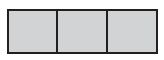•  Equal parts: Name:
• Question 2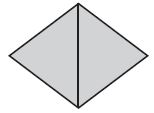•  Equal parts: Name:
• Question 3

Diego cuts a round pizza into eight equal slice. What is the name for the parts?

Write whether the shape is divided into equal parts or unequal parts.

• Question 4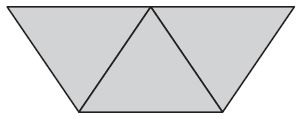•  parts
• Question 5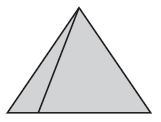•  parts
• Question 6

Two friends share 3 fruit bars equally. How much does each friend get?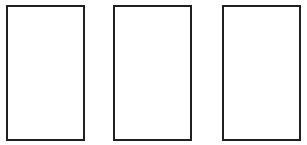• 1 half
• 2 thirds
• 2 halves
• 3 halves
• Question 7

Four brothers share 3 pizzas equally. How much of a pizza does each brother get?• 3 halves
• 4 third
• 3 fourths
• 2 fourths
• Question 8

Tyler made a pan of cornbread. He cut it into 8 equal pieces and ate 1 piece. What fraction of the cornbread did Tyler eat?

• Question 9

Anna cut an apple into 4 equal pieces. She gave 1 piece to her sister. What fraction of the apple did Anna give to her sister?

• Question 10

What fraction names the shaded part?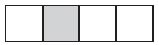• $$\large \frac{1}{3}$$
• $$\large \frac{1}{4}$$
• $$\large \frac{1}{6}$$
• $$\large \frac{1}{8}$$

Yes, email page to my online tutor.